# KVPY-SX 2019 Chemistry Paper with Solutions

The Department of Technology & Science, Government of India conducts the KVPY exam to inspire the students to take up basic science courses and research careers in science by giving scholarships. KVPY-SX 2019 Chemistry paper solutions are given on this page. BYJU’S provides step by step solutions so that students can easily understand them. Students are advised to revise these solutions so that they will get an idea of the exam pattern and difficulty level.

### KVPY SX 2019 - Chemistry

Question 1: The major product of the following reaction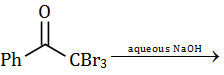are: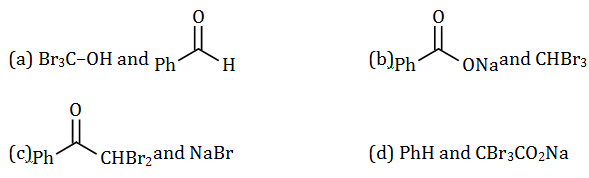Solution: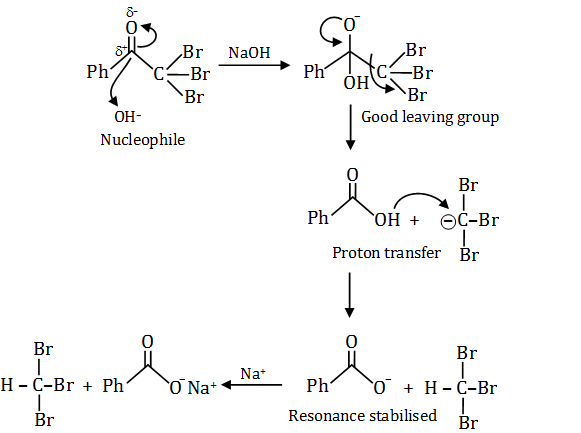Option (b) is correct.

Question 2: Among the following,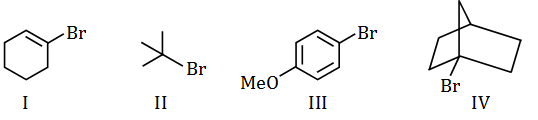The compounds which can undergo an SN1 reaction in an aqueous solution, are

1. (a) I and IV only
2. (b) II and IV only
3. (c) II and III only
4. (d) II, III and IV only

Solution: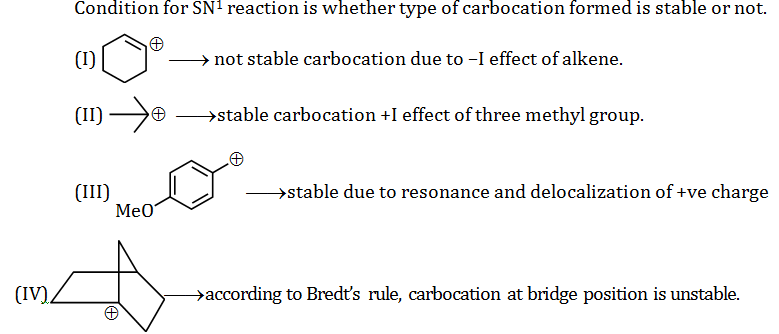So, option (c) is correct.

Question 3: The major product of the following reaction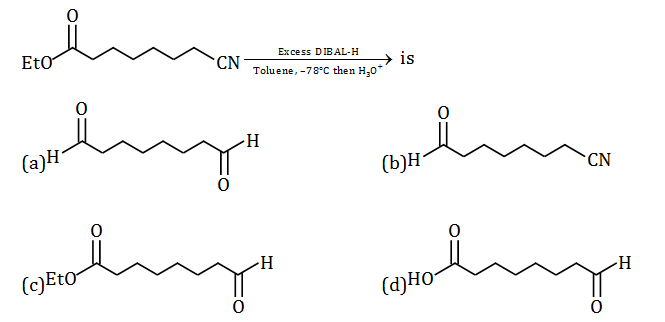Solution:

[DIBAL–H] -> (i–Bu)2 Al–H -> Di isobutyl aluminium hydride.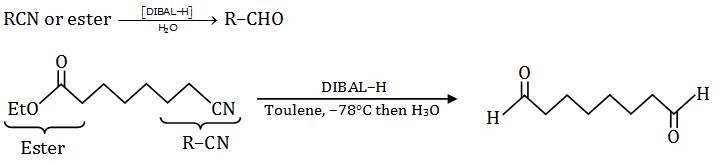Question 4: Permanent hardness of water can be removed by

1. (a) heating
2. (b) treating with sodium acetate (CH3CO2Na)
3. (c) treating with Ca(HCO3)2
4. (d) treatment with sodium hexametaphosphate (Na6P6O18)

Solution:

The permanent hardness of water can be removed by using calgon process. The hardness of water causes by salts of Ca and Mg.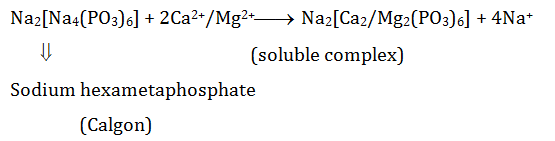Question 5: Alkali metals (M) dissolve in liquid NH3 to give

1. (a) MNH2
2. (b) MH
3. (c) [M(NH3)x]+ + [e(NH3)y]
4. (d) M3N

Solution:

All alkali metals like lithium, sodium, potassium etc. dissolves in liquid ammonia to give deep blue coloured solution. The blue colour is due to presence of solvated electrons.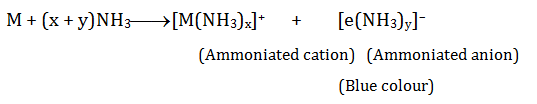Question 6: The absolute configurations of the following compounds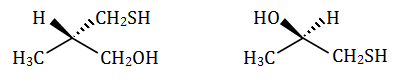Respectively are

1. (a) R and R
2. (b) S and S
3. (c) R and S
4. (d) S and R

Solution:

Draw the Fischer project i.e. 2–D representation and then assign priorities.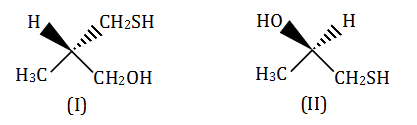According to Cahn Ingold Prelog (CIP) priority rules –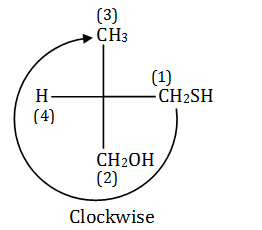So, it should be ‘R’ but here, lower priority group is on horizontal line. Therefore, Configuration will be reversed. i.e. correct configuration ‘S’.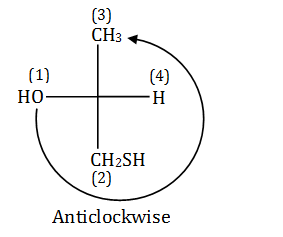It should be ‘S’ lower priority group is on

horizontal line i.e. ‘R’.

So, option (d), S, R is correct.

Question 7: The diamagnetic species among the following is

1. (a) O2+
2. (b) O2-
3. (c) O2
4. (d) O22-

Solution:

According to MOT –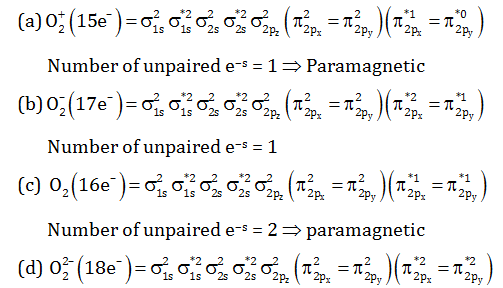Number of unpaired e–s = 0 => diamagnetic

Option (d) is correct.

Question 8: Among the following transformations, the hybridization of the central atom remains unchanged in

1. (a) CO2 -> HCOOH
2. (b) BF3 -> BF4-
3. (c) NH3-> NH4+
4. (d) PCl3 -> PCl5

Solution: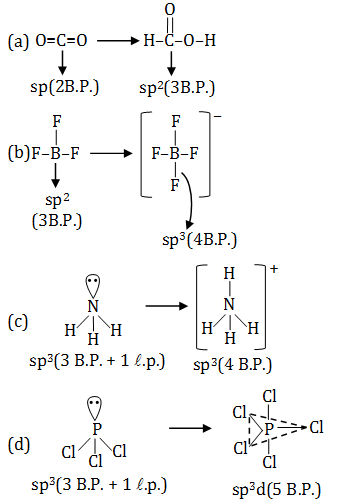Question 9: For an octahedral complex MX4Y2 (M = a transition metal, X and Y are monodentate achiral ligands), the correct statement, among the following, is

1. (a) MX4Y2 has 2 geometrical isomers one of which is chiral
2. (b) MX4Y2 has 2 geometrical isomers both of which are achiral
3. (c) MX4Y2 has 4 geometrical isomers all of which are achiral
4. (d) MX4Y2 has 4 geometrical isomers two of which are chiral

Solution:

MX4Y2-> Octahedral complex

Note -> If there is plane of symmetry (POS) or centre of symmetry (COS) in a complex. Then it will be achiral and optically inactive. Geometrical isomers exist is cis and trans forms.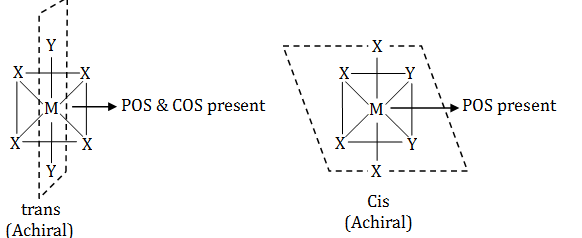i.e. two geometrical isomers both of which are achiral.

So, option (b) is correct.

Question 10: The values of the Henry's law constant of Ar, CO2, CH4 and O2 in water at 250C are 40.30, 1.67, 0.41 and 34.86 kbar, respectively. The order of their solubility in water at the same temperature and pressure is

1. (a) Ar > O2> CO2> CH4
2. (b) CH4> CO2> Ar > O2
3. (c) CH4> CO2> O2> Ar
4. (d) Ar > CH4> O2> CO2

Solution:

According to Henry’s law, the partial pressure of a gas in vapour phase (P) is proportional to the mole fraction of the gas (X) in the solution.

P proportional to X

P = KHX

KH = Henry’s constant

P = Partial pressure of gas above liquid surface

so, according to Henry’s law solubility of gas in liquid proportional to partial pressure of gas above liquid surface.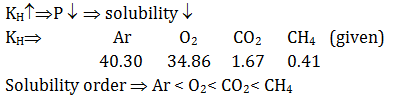Question 11: Thermal decomposition of N2O5 occurs as per the equation below

2N2O5-> 4NO2 + O2

The correct statement is

1. (a) O2 production rate is four times the NO2 production rate
2. (b) O2 production rate is the same as the rate of disappearance of N2O5
3. (c) rate of disappearance of N2O5 is one-fourth of NO2 production rate
4. (d) rate of disappearance of N2O5 is twice the O2 production rate.

Solution: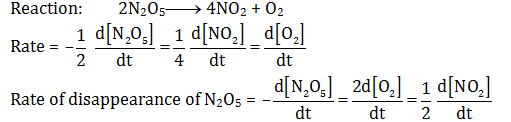Rate of disappearance of N2O5= twice the O2 production rate

So, option (d) is correct.

Question 12: For a 1st order chemical reaction.

1. (a) the product formation rate is independent of reactant concentration
2. (b) the time taken for the completion of half of the reaction (t1/2) is 69.3% of the rate constant (k)
3. (c) the dimension of Arrhenius pre-exponential factor is reciprocal of time
4. (d) the concentration vs time plot for the reactant should be linear with a negative slope.

Solution:

For Ist order reaction –

t = (2.303/K) log10 [A0/At]

And t1/2 = 0.693/K

Arrhenius equation: K = Ae-Ea/RT

[A = pre-exponential factor]

In a first order reaction, unit of rate constant is s–1. So pre-exponential factor are also reciprocal seconds. Because exponential factor depends on frequency of collision. Its related to collision theory and transition state theory.

Question 13: The boiling point of 0.001 M aqueous solutions of NaCl, Na2SO4, K3PO4 and CH3COOH should follows the order

1. (a) CH3COOH < NaCl < Na2SO4< K3PO4
2. (b) NaCl < Na2SO4< K3PO4< CH3COOH
3. (c) CH3COOH < K3PO4< Na2SO4< NaCl
4. (d) CH3COOH < K3PO4< NaCl < Na2SO4

Solution:

We know that, ∆Tb = i ×Kb × m

molarity or molality = 0.001 m {at very diluted solution}

So, ∆Tb proportional to i ;{molality is constant for all}

i increases as Boiling point increases

For NaCl => strong electrolyte i = 2 {Na+ + Cl}

For Na2SO4=> strong electrolyte i = 3 {2Na+ + SO42–}

For K3PO4=> strong electrolyte i = 4 {3K++PO43-}

For CH3COOH => weak acid i < 2 (calculated from i= 1+ (n-1)α , where α<1 )

So, order of Boiling Point: K3PO4> Na2SO4> NaCl > CH3COOH

So, option (a) is correct.

Question 14: An allotrope of carbon which exhibits only two types of C–C bond distance of 143.5 pm and 138.3 pm, is

1. (a) charcoal
2. (b) graphite
3. (c) diamond
4. (d) fullerene

Solution:

Fullerene a soccer ball shaped molecule has 60 vertices with a carbon atom at each vertex. It contains both single and double bond with C–C at a distance of 143.5 pm and 138.3 pm.

Where as in diamond C–C bond length 154 pm and in graphite 140 pm.

Question 15: Nylon-2-nylon-6 is a co-polymer of 6-aminohexanoic acid and

1. (a) glycine
2. (b) valine
3. (c) alanine
4. (d) leucine

Solution:

Nylon–2–Nylon–6 is a copolymer of glycine and amino caproic acid (6-amino hexanoic acid)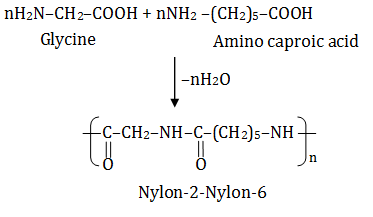Question 16: A solid is hard and brittle. It is an insulator solid state but conducts electricity in molten state. The solid is a

1. (a) molecular solid
2. (b) ionic solid
3. (c) metallic solid
4. (d) covalent solid

Solution:

Ionic solid is hard and brittle eg. NaCl

It is an insulator in solid state but in molten state it conducts electricity as it has free ions in molten state.

Question 17: The curve that best describes the adsorption of a gas (x g) on 1.0 g of a solid substrate as a function of pressure (p) at a fixed temperature is: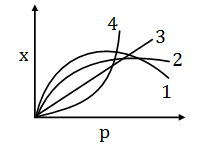1. (a) 1
2. (b) 2
3. (c) 3
4. (d) 4

Solution:

Given: mass = 1g

x/m = KP1/n (n>1)

x = KP1/n

x/K = P1/n

(x/K)n = P

On comparing parabola equation yn = x. If n = 2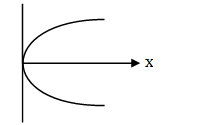So, graph (b) is correct.

Question 18: The octahedral complex CoSO4Cl.5NH3 exists in two isomeric forms X and Y. Isomer X reacts with AgNO3 to give a white precipitate, but does not react with BaCl2, Isomer Y gives white precipitate with BaCl2 but does react with AgNO3.

Isomers X and Y are

1. (a) Ionization isomers
3. (c) Coordination isomers
4. (d) Solvate isomers

Solution:

CoSO4Cl.5NH3

Isomers of CoSO4Cl.5NH3are (I) [Co(NH3)5SO4]Cl gives white ppt with AgNO3 but not with BaCl2 and (II) [Co(NH3)5Cl]SO4 gives white ppt with BaCl2 but not with AgSO4.

(I) [Co(NH3)5SO4]Cl + AgNO3 -> AgCl + [Co(NH3)5SO4]NO3

[white ppt]

(II) [Co(NH3)5Cl]SO4 + BaCl2 -> BaSO4 + [Co(NH3)5Cl]Cl2

[white ppt]

So, isomer (I) and (II) are ionization isomers.

Question 19: The correct order of basicity of the following amines is: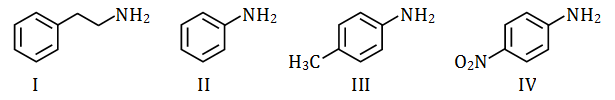1. (a) I > II > III > IV
2. (b) I > III > II > IV
3. (c) III > II > I > IV
4. (d) IV > III > II > I

Solution: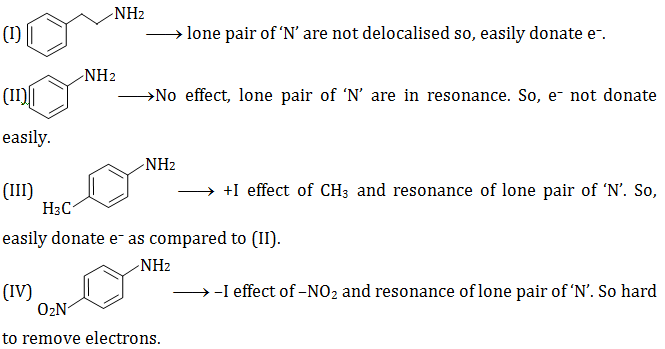So, order of basicity - I > III > II > IV

Question 20: Electrolysis of a concentrated aqueous solution of NaCl results in

1. (a) increase in pH of the solution
2. (b) decrease in pH of the solution
3. (c) O2 liberation at the cathode
4. (d) H2 liberation at the anode

Solution:

Electrolysis of aqueous NaCl leads to the formation of NaOH, which is a base. So, its pH will be increases.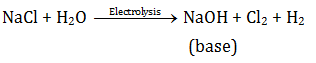H2 liberation will be at cathode due to (H+-> H2) gain of electron at cathode.

So, option (a) is correct.

Question 21: The product of which of the following reaction forms a reddish brown precipitate when subjected to Fehling's test?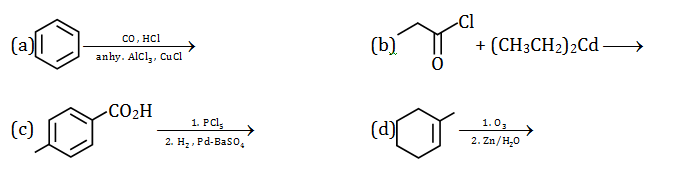Solution: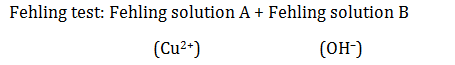Fehling reagent react with aldehyde which has α–H such aldehyde form an enolate and thus give a positive Fehling test and a reddish brown precipitate is obtained.

Reaction: R–CHO + 2Cu2++ 5OH ->RCOO + Cu2O + 3H2O (reddish brown)

Benzaldehyde gives tollen’s as well as schiff’s test but not gives fehling's solution test because benzaldehyde does not contain Alpha hydrogen and cannot form intermediate enolate to proceed for further and hence it does not react with fehling's solution test however aliphatic aldehydes gives fehling's solution test.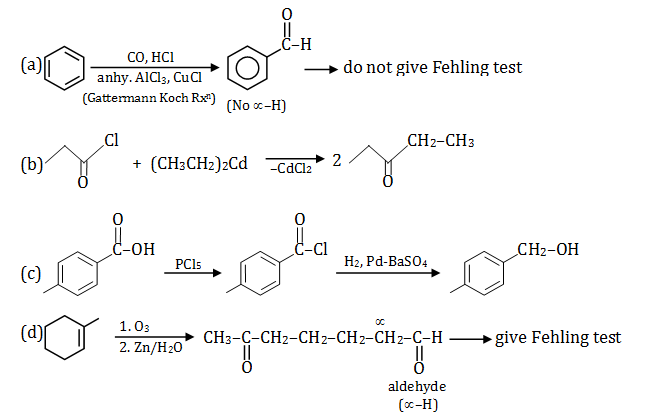So, option (d) product gives Fehling test.

Question 22: The major products X, Y and Z in the following sequence of transformations are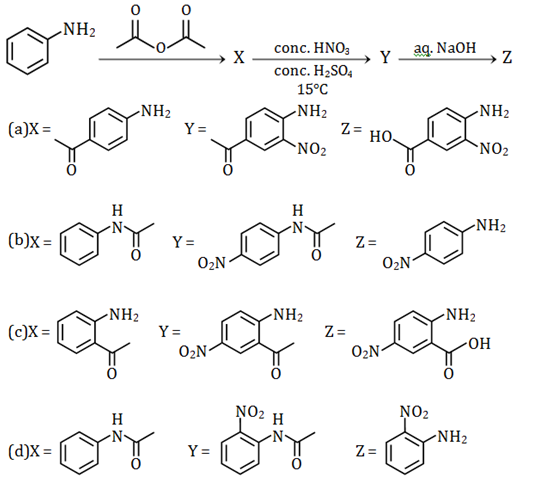Solution: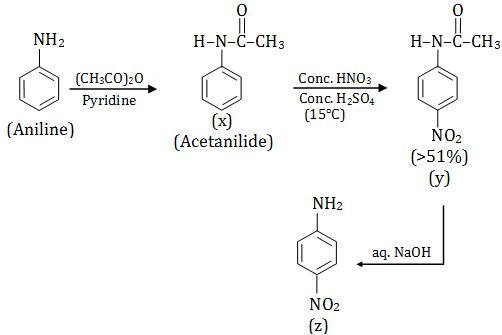So, option (b) is correct.

Question 23: In the following reaction, P gives two products Q and R, each in 40% yield.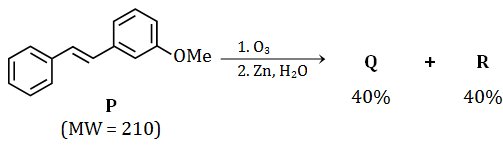If the reaction is carried out with 420 mg of P, the reaction yields 108.8 mg of Q.

The amount of R produced in the reaction is closest to

1. (a) 97.6 mg
2. (b) 108.8 mg
3. (c) 84.8 mg
4. (d) 121.6 mg

Solution: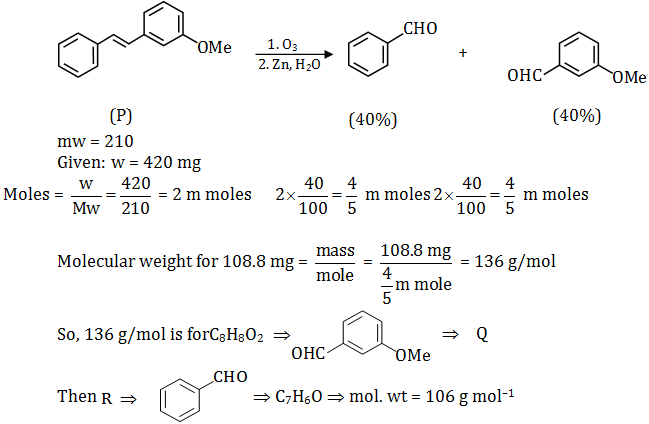Mass of R = mole × m.wt. = (4/5 )m mole × 106 g mol–1 = 84.4 mg

So, option (c) is correct.

Question 24: Solubility products of CuI and Ag2CrO4 have almost the same value (~4 × 10–12).

The ratio of solubilities of the two salts (CuI: Ag2CrO4) is closest to

1. (a) 0.01
2. (b) 0.02
3. (c) 0.03
4. (d) 0.10

Solution: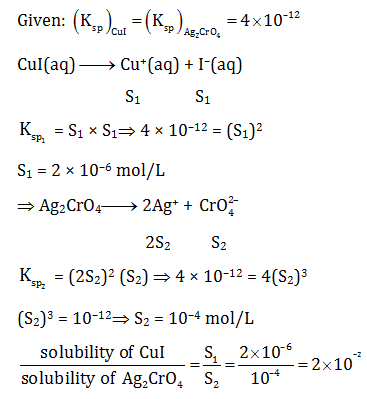S1/S2 = 0.02

So, option (b) is correct.

Question 25: Given that the molar combustion enthalpy of benzene, cyclohexane, and hydrogen are x, y, and z, respectively, the molar enthalpy of hydrogenation of benzene to cyclohexane is

1. (a) x – y + z
2. (b) x – y + 3z
3. (c) y – x + z
4. (d) y – x + 3z

Solution: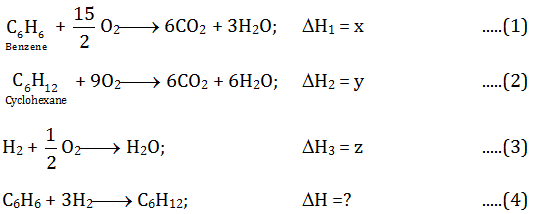So, equation (1) + equation (3) × 3 – equation (2); to get equation (4)

C6H6 + 3H2 -> C6H12

x + 3z – y = x – y + 3z

So, molar enthalpy of hydrogenation of benzene to cyclo hexane = x – y + 3z

Option (b) is correct.

Question 26: Among the following, the pair of paramagnetic complexes is

1. (a) K3[Fe(CN)6] and K3[CoF6]
2. (b) K3[Fe(CN)6] and [Co(NH3)6]Cl3
3. (c) K4[Fe(CN)6] and K3[CoF6]
4. (d) K4[Fe(CN)6] and [Co(NH3)6]Cl3

Solution: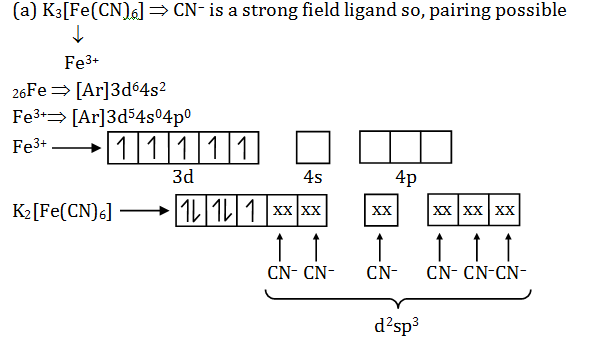Number of unpaired e–s = 1 => paramagnetic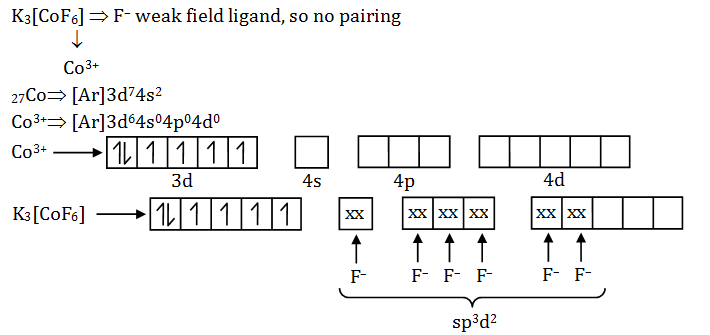Number of unpaired e–s = 4 => paramagnetic

So, option (a) is correct.

Question 27: The major products X and Y in the following sequence of transformations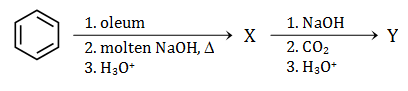are: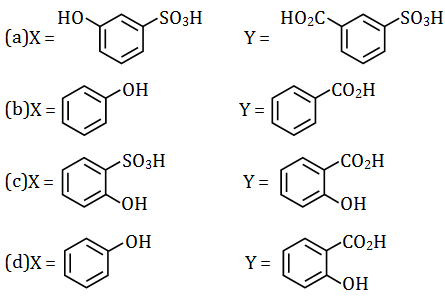Solution: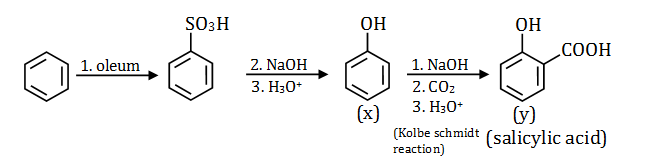So, option (d) is correct.

Question 28: 3.0 g of oxalic acid [(CO2H)2.2H2O] is dissolved in a solvent to prepare a 250 mL solutions. The density of the solution is 1.9 g/mL. The molality and normality of the solution, respectively, are closest to

1. (a) 0.10 and 0.38
2. (b) 0.10 and 0.19
3. (c) 0.05 and 0.19
4. (d) 0.05 and 0.09

Solution:

Given: Mass of oxalic acid = 3.0 g

Mol. wt. of oxalic acid (COOH)2.2H2O = 126 g mol–1

Density = 1.9 g/mL and volume of solution = 250 mL

d = m/v

m = d × v

Molality = mass of solute/m.mass of solute× Mass of solvent(kg)

Molality = m/m.m×(d×v) = 3/(126×10.9×250×10-3)

Molality = 0.05

We know normality = molality ×nfactor

Molarity = mole/volume(L)

nfactor of oxalic acid = 2 (because it lose 2H+)

N = (n/VL) ×nfactor

Normality = ((3/126)/(250 ×10-3))2

= 0.19

So, option (c) is correct.

Question 29: In a titration experiment, 10 mL of a FeCl2 solution consumed 25 mL of a standard K2Cr2O7 solution to reach the equivalent point. The standard K2Cr2O7 solution is prepared by dissolving 1.225 g of K2Cr2O7 in 250 mL water. The concentration of the FeCl2 solution is closest to [Given: molecular weight of K2Cr2O7 = 294 g mol–1]

1. (a) 0.25 N
2. (b) 0.50 N
3. (c) 0.10 N
4. (d) 0.04 N

Solution: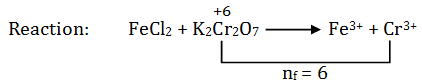So, by law of equivalence,

Number of eq. of FeCl2 = number of eq. of K2Cr2O7

N1V1 = N2V2

Given: volume of FeCl2 = 10 mL

Volume of K2Cr2O7 = 25 mL

Wt. of K2Cr2O7 = 1.225 g

Volume of solution = 250 mL

m. wt. of K2Cr2O7 = 294 g mol–1

N1×10 = M×nf ×25

N1×10 = ((1.225/294)/(250×10-3))25×6

Molarity = mole/volume(L)

N1 = 1.225×25×6/(294×250×10-3×10)

= 0.25N

Question 30: Atoms of an element Z form hexagonal closed pack (hcp) lattice and atoms of element X occupy all the tetrahedral voids. The formula of the compound is

1. (a) XZ
2. (b) XZ2
3. (c) X2Z
4. (d) X4Z3

Solution:

Let, number of atom in HCP = a

We know, the number of tetrahedral voids will be twice as that of number of atoms present in a unit cell.

So, no. of tetrahedral void = 2a

Z = a, X = 2a

Z : X

a : 2a

1 : 1

So, formula of compound = X2Z

Therefore, option (c) is correct.

### KVPY-SX 2019 Chemistry Paper with Solutions